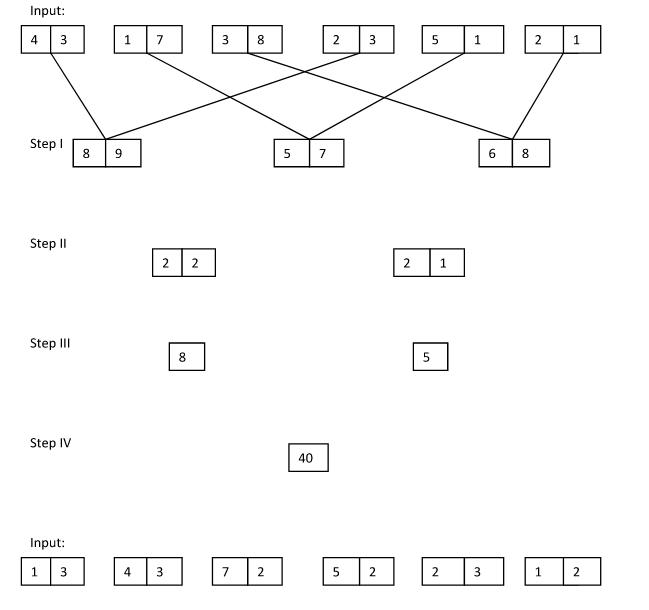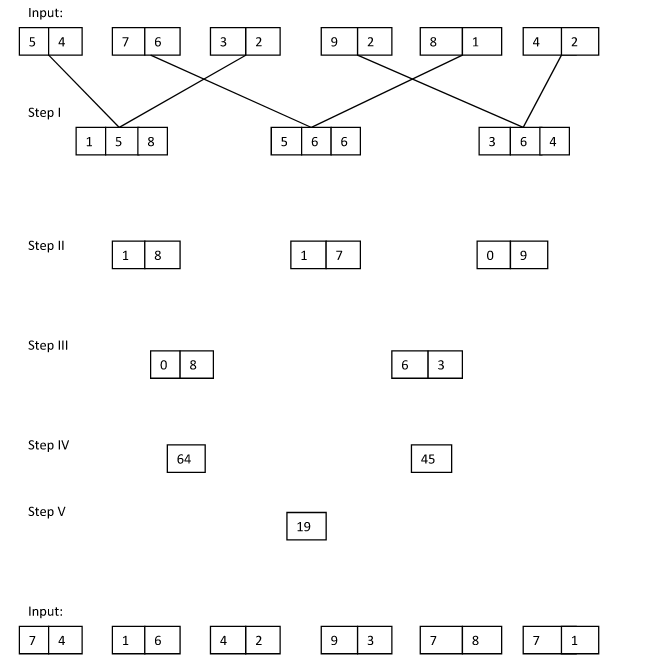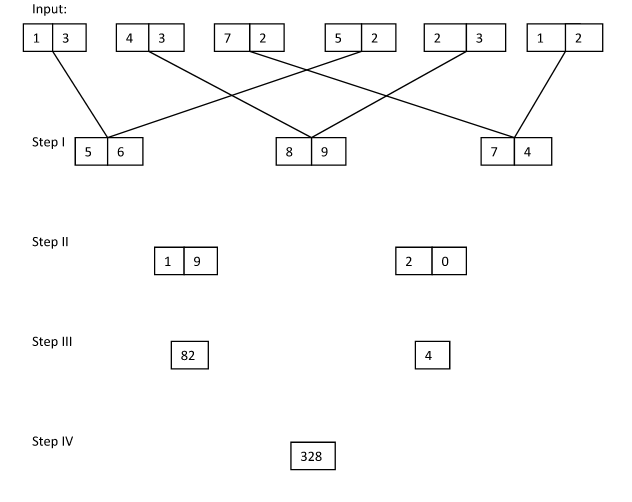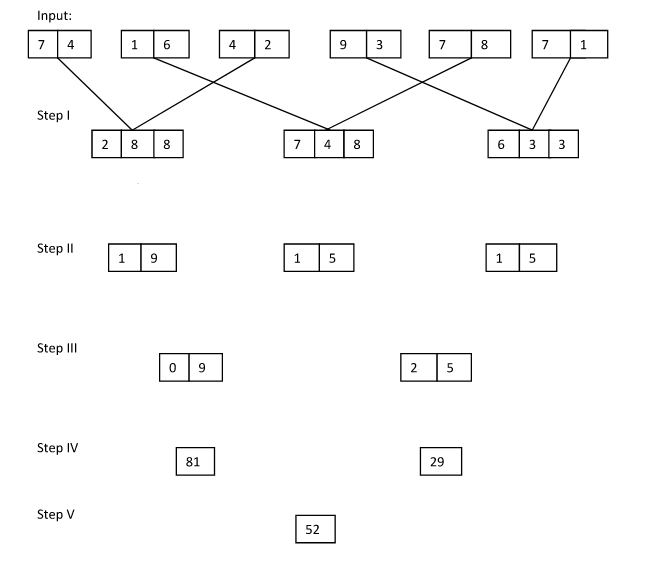# Crack SBI Clerk 2018 – Logical Reasoning (New Pattern Input Output) Day-25

## Crack SBI Clerk 2018 – Logical Reasoning New Pattern (New Pattern Input Output) Day-25:

Dear Readers, Reasoning Section play a vital role in Banking and all other competitive exams. This year 2018 was started with three wonderful opportunities; Most Awaited SBI Clerk 2018. To enrich your preparation here we have providing new series of Practice Questions on Reasoning Ability – Input Output New Pattern. Candidates those who are going to appear in Bank Exams 2018 can practice these questions daily and make your preparation effective.

[WpProQuiz 1435]

Click “Start Quiz” to attend these Questions and view Solutions

## Daily Practice Test Schedule | Good Luck

 Topic Daily Publishing Time Daily News Papers & Editorials 8.00 AM Current Affairs Quiz 9.00 AM Logical Reasoning 10.00 AM Quantitative Aptitude “20-20” 11.00 AM Vocabulary (Based on The Hindu) 12.00 PM Static GK Quiz 1.00 PM English Language “20-20” 2.00 PM Banking Awareness Quiz 3.00 PM Reasoning Puzzles & Seating 4.00 PM Daily Current Affairs Updates 5.00 PM Data Interpretation / Application Sums (Topic Wise) 6.00 PM Reasoning Ability “20-20” 7.00 PM English Language (New Pattern Questions) 8.00 PM General / Financial Awareness Quiz 9.00 PM

Directions (1-5): Study the following information carefully and answer the given questions.

A number arrangement machine arranges two digit numbers into a typical manner. Each step gives output taking input from the previous step. Thefollowing is an illustration of Input and rearrangement. Using the illustration answer the question given below.1.Which of the following combination represent the first digit of the third number and second digit of the first number in step I of the given input?

a) 9, 5

b) 7, 6

c) 4, 8

d) 4, 7

e) None of these

1. If each digit in the step II is doubled and then added, then what will be the final sum?

a) 32

b) 36

c) 20

d) 24

e) None of these

3.What is the multiplication of two numbers obtained in step II?

a) 380

b) 190

c) 360

d) 450

e) None of these

4.If the value ‘6^3’ is subtracted from the final output, then what will be the resultant value?

a) 120

b) 116

c) 144

d) 162

e) None of these

5.Which of the following represent the difference between the first digit of the second number and second digit of the first number in step II?

a) 5

b) 6

c) 7

d) 3

e) None of these

Directions (6-10): Study the following information carefully and answer the given questions.

A number arrangement machine arranges two digit numbers into a typical manner. Each step gives output taking input from the previous step. The following is an illustration of Input and rearrangement. Using the illustration answer the question given below.1. If the value ‘48’ is added to the final output, then what will be the resultant value?

a) 80

b) 90

c) 100

d) 120

e) None of these

7.Which of the following represent the difference between the first digit of the second number and second digit of the third number in step I?

a) 4

b) 5

c) 6

d) 8

e) None of these

1. If each digit in the step II is halved and then added, then what will be the final sum?

a) 15

b) 11

c) 17

d) 19

e) None of these

9.Which of the following combination represent the first digit of the third number and second digit of the first number in step II of the given input?

a) 5, 1

b) 1, 5

c) 9, 5

d) 1, 9

e) None of these

10.What is the multiplication of two numbers obtained in step III?

a) 175

b) 325

c) 250

d) 425

e) None of these

Direction (1-5)Step I – First digit multiply with the first digit and the second digit multiply with the second digit

Step II –

First number – Sum of first three digits–5 + 6 + 8 = 19

Second number – Sum of last three digits –9 + 7 + 4 = 20

Step III – Sum of squares of both digits within the number

First number – 81 + 1 = 82

Second number – 4 + 0 = 4

Step IV – Multiplication of both numbers

Directions (6-10):Step I – First digit multiply with the first digit and the second digit multiply with the second digit

Step II –

First number – Sum of the third digit of all three numbers – 8 + 8 + 3 = 19

Second number – Sum of the second digit of all three numbers – 8 + 4 + 3 = 15

Third number – Sum of the first digit of all three numbers – 2 + 7 + 6 = 15

Step III –

First number – Multiplication of first three numbers – 1 × 9 × 1 = 09

Second number – Multiplication of last three numbers – 5 × 1 × 5 = 25

Step IV – Sum of the squares of the number

First number = 0^2 + 9^2 = 81

Second number = 2^2 + 5^2 = 29

Step V –Difference of both numbers